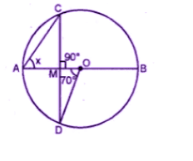Guru

# If O is the centre of the circle, find the value of x in each of the following figures (using the given information):

• 2

This is an important and exam oriented question from Chapter name- circles
Topic – Angle properties of circles
Chapter number- 15

ICSEIn this question we have figure of circles with constructions and we have to find the value of x using basic properties of circles.

Avichal publication
Understanding ICSE Mathematics
Question2

Share

1. Arc AB subtends ∠AOD at the centre and ∠ACD at the remaining part of the Circle

∠AOD = 2 ∠ACB

∠ACB = ½ ∠AOD = ½ × 70= 35o

∠CMO = 90o

∠AMC = 90o

(∠AMC + ∠CMO = 180o)Now in ∆ACM

∠ACM + ∠AMC + ∠CAM = 180o

35o + 90+ x= 180o

125+ x= 180o

X= 180 – 125= 55o

• 0